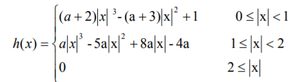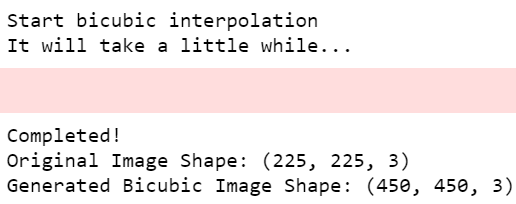Related Articles

# Python OpenCV – Bicubic Interpolation for Resizing Image

• Difficulty Level : Expert
• Last Updated : 08 May, 2021

Image resizing is a crucial concept that wishes to augment or reduce the number of pixels in a picture. Applications of image resizing can occur under a wider form of scenarios: transliteration of the image, correcting for lens distortion, changing perspective, and rotating a picture. The results of resizing greatly vary looking on the kind of interpolation algorithm used.

Note: While applying interpolation algorithms, some information is certain to be lost as these are approximation algorithms.

## What is Interpolation?

Interpolation works by using known data to estimate values at unknown points. For example: if you wanted to understand the pixel intensity of a picture at a selected location within the grid (say coordinate (x, y), but only (x-1,y-1) and (x+1,y+1) are known, you’ll estimate the value at (x, y) using linear interpolation. The greater the quantity of already known values, the higher would be the accuracy of the estimated pixel value.

## Interpolation Algorithms

Different interpolation algorithms include the nearest neighbor, bilinear, bicubic, and others. Betting on their complexity, these use anywhere from 0 to 256 (or more) adjacent pixels when interpolating. The accuracy of those algorithms is increased significantly by increasing the number of neighboring pixels considered while evaluation of the new pixel value. Interpolation algorithms are predominantly used for resizing and distorting a high-resolution image to an occasional resolution image. There are various interpolation algorithms one of them is Bicubic Interpolation.

## Bicubic Interpolation

In addition to going 2×2 neighborhood of known pixel values, Bicubic goes one step beyond bilinear by considering the closest 4×4 neighborhood of known pixels — for a complete of 16 pixels. The pixels that are closer to the one that’s to be estimated are given higher weights as compared to those that are further away. Therefore, the farthest pixels have the smallest amount of weight. The results of Bicubic interpolation are far better as compared to NN or bilinear algorithms. This can be because a greater number of known pixel values are considered while estimating the desired value. Thus, making it one of all the foremost standard interpolation methods.

### Implementing Bicubic Interpolation with Python

Importing the necessary modules: We import all dependencies like cv2 (OpenCV), NumPy, and math.

## Python

 `# Import modules``import` `cv2``import` `numpy as np``import` `math``import` `sys, time`

Writing the Interpolation Kernel Function for Bicubic Interpolation: The interpolation kernel for bicubic is of the form:Kernel equation

Here the value of coefficient a determines the performance of the kernel and it lies mostly between -0.5 to -0.75 for optimum performance.

## Python

 `# Interpolation kernel``def` `u(s, a):``   ` `    ``if` `(``abs``(s) >``=` `0``) & (``abs``(s) <``=` `1``):``        ``return` `(a``+``2``)``*``(``abs``(s)``*``*``3``)``-``(a``+``3``)``*``(``abs``(s)``*``*``2``)``+``1``       ` `    ``elif` `(``abs``(s) > ``1``) & (``abs``(s) <``=` `2``):``        ``return` `a``*``(``abs``(s)``*``*``3``)``-``(``5``*``a)``*``(``abs``(s)``*``*``2``)``+``(``8``*``a)``*``abs``(s)``-``4``*``a``    ``return` `0`

Adding padding to the input image: Define padding function to add borders to your image. OpenCV has various padding functions. When interpolations require padding the source, the boundary of the source image needs to be extended because it needs to have information such that it can compute the pixel values of all destination pixels that lie along the boundaries.

## Python

 `# Padding``def` `padding(img, H, W, C):``    ``zimg ``=` `np.zeros((H``+``4``, W``+``4``, C))``    ``zimg[``2``:H``+``2``, ``2``:W``+``2``, :C] ``=` `img``     ` `    ``# Pad the first/last two col and row``    ``zimg[``2``:H``+``2``, ``0``:``2``, :C] ``=` `img[:, ``0``:``1``, :C]``    ``zimg[H``+``2``:H``+``4``, ``2``:W``+``2``, :] ``=` `img[H``-``1``:H, :, :]``    ``zimg[``2``:H``+``2``, W``+``2``:W``+``4``, :] ``=` `img[:, W``-``1``:W, :]``    ``zimg[``0``:``2``, ``2``:W``+``2``, :C] ``=` `img[``0``:``1``, :, :C]``     ` `    ``# Pad the missing eight points``    ``zimg[``0``:``2``, ``0``:``2``, :C] ``=` `img[``0``, ``0``, :C]``    ``zimg[H``+``2``:H``+``4``, ``0``:``2``, :C] ``=` `img[H``-``1``, ``0``, :C]``    ``zimg[H``+``2``:H``+``4``, W``+``2``:W``+``4``, :C] ``=` `img[H``-``1``, W``-``1``, :C]``    ``zimg[``0``:``2``, W``+``2``:W``+``4``, :C] ``=` `img[``0``, W``-``1``, :C]``     ` `    ``return` `zimg`

Writing the bicubic interpolation function: Define bicubic function and pass the image as an input. (You can vary the scaling factor as x2 or x4 based on the requirement.)

## Python

 `# Bicubic operation``def` `bicubic(img, ratio, a):`` ` `    ``# Get image size``    ``H, W, C ``=` `img.shape`` ` `    ``# Here H = Height, W = weight,``    ``# C = Number of channels if the``    ``# image is coloured.``    ``img ``=` `padding(img, H, W, C)`` ` `    ``# Create new image``    ``dH ``=` `math.floor(H``*``ratio)``    ``dW ``=` `math.floor(W``*``ratio)`` ` `    ``# Converting into matrix``    ``dst ``=` `np.zeros((dH, dW, ``3``))`` ` `    ``# np.zeroes generates a matrix``    ``# consisting only of zeroes``    ``# Here we initialize our answer``    ``# (dst) as zero`` ` `    ``h ``=` `1``/``ratio`` ` `    ``print``(``'Start bicubic interpolation'``)``    ``print``(``'It will take a little while...'``)``    ``inc ``=` `0`` ` `    ``for` `c ``in` `range``(C):``        ``for` `j ``in` `range``(dH):``            ``for` `i ``in` `range``(dW):`` ` `                ``# Getting the coordinates of the``                ``# nearby values``                ``x, y ``=` `i ``*` `h ``+` `2``, j ``*` `h ``+` `2`` ` `                ``x1 ``=` `1` `+` `x ``-` `math.floor(x)``                ``x2 ``=` `x ``-` `math.floor(x)``                ``x3 ``=` `math.floor(x) ``+` `1` `-` `x``                ``x4 ``=` `math.floor(x) ``+` `2` `-` `x`` ` `                ``y1 ``=` `1` `+` `y ``-` `math.floor(y)``                ``y2 ``=` `y ``-` `math.floor(y)``                ``y3 ``=` `math.floor(y) ``+` `1` `-` `y``                ``y4 ``=` `math.floor(y) ``+` `2` `-` `y`` ` `                ``# Considering all nearby 16 values``                ``mat_l ``=` `np.matrix([[u(x1, a), u(x2, a), u(x3, a), u(x4, a)]])``                ``mat_m ``=` `np.matrix([[img[``int``(y``-``y1), ``int``(x``-``x1), c],``                                    ``img[``int``(y``-``y2), ``int``(x``-``x1), c],``                                    ``img[``int``(y``+``y3), ``int``(x``-``x1), c],``                                    ``img[``int``(y``+``y4), ``int``(x``-``x1), c]],``                                   ``[img[``int``(y``-``y1), ``int``(x``-``x2), c],``                                    ``img[``int``(y``-``y2), ``int``(x``-``x2), c],``                                    ``img[``int``(y``+``y3), ``int``(x``-``x2), c],``                                    ``img[``int``(y``+``y4), ``int``(x``-``x2), c]],``                                   ``[img[``int``(y``-``y1), ``int``(x``+``x3), c],``                                    ``img[``int``(y``-``y2), ``int``(x``+``x3), c],``                                    ``img[``int``(y``+``y3), ``int``(x``+``x3), c],``                                    ``img[``int``(y``+``y4), ``int``(x``+``x3), c]],``                                   ``[img[``int``(y``-``y1), ``int``(x``+``x4), c],``                                    ``img[``int``(y``-``y2), ``int``(x``+``x4), c],``                                    ``img[``int``(y``+``y3), ``int``(x``+``x4), c],``                                    ``img[``int``(y``+``y4), ``int``(x``+``x4), c]]])``                ``mat_r ``=` `np.matrix(``                    ``[[u(y1, a)], [u(y2, a)], [u(y3, a)], [u(y4, a)]])``                 ` `                ``# Here the dot function is used to get the dot ``                ``# product of 2 matrices``                ``dst[j, i, c] ``=` `np.dot(np.dot(mat_l, mat_m), mat_r)`` ` `    ``# If there is an error message, it``    ``# directly goes to stderr``    ``sys.stderr.write(``'\n'``)``     ` `    ``# Flushing the buffer``    ``sys.stderr.flush()``    ``return` `dst`

Taking input from the user and passing the input to the bicubic function to generate the resized image: Passing the desired image to the bicubic function and saving the output as a separate file in the directory.

## Python3

 `# Read image``# You can put your input image over here ``# to run bicubic interpolation``# The read function of Open CV is used ``# for this task``img ``=` `cv2.imread(``'gfg.png'``)`` ` `# Scale factor``ratio ``=` `2`` ` `# Coefficient``a ``=` `-``1``/``2`` ` `# Passing the input image in the ``# bicubic function``dst ``=` `bicubic(img, ratio, a)  ``print``(``'Completed!'``)`` ` `# Saving the output image``cv2.imwrite(``'bicubic.png'``, dst) ``bicubicImg``=``cv2.imread(``'bicubic.png'``)`

Compare the generated image with the input image: Use the shape() method to compare the height, width, and color mode of both images.

## Python3

 `# display shapes of both images``print``(``'Original Image Shape:'``,img.shape)``print``(``'Generated Bicubic Image Shape:'``,bicubicImg.shape)`

Complete Code:

Input Image:gfg.png

## Python3

 `# import modules``import` `cv2``import` `numpy as np``import` `math``import` `sys``import` `time`` ` ` ` `# Interpolation kernel``def` `u(s, a):``    ``if` `(``abs``(s) >``=` `0``) & (``abs``(s) <``=` `1``):``        ``return` `(a``+``2``)``*``(``abs``(s)``*``*``3``)``-``(a``+``3``)``*``(``abs``(s)``*``*``2``)``+``1``    ``elif` `(``abs``(s) > ``1``) & (``abs``(s) <``=` `2``):``        ``return` `a``*``(``abs``(s)``*``*``3``)``-``(``5``*``a)``*``(``abs``(s)``*``*``2``)``+``(``8``*``a)``*``abs``(s)``-``4``*``a``    ``return` `0`` ` ` ` `# Padding``def` `padding(img, H, W, C):``    ``zimg ``=` `np.zeros((H``+``4``, W``+``4``, C))``    ``zimg[``2``:H``+``2``, ``2``:W``+``2``, :C] ``=` `img``     ` `    ``# Pad the first/last two col and row``    ``zimg[``2``:H``+``2``, ``0``:``2``, :C] ``=` `img[:, ``0``:``1``, :C]``    ``zimg[H``+``2``:H``+``4``, ``2``:W``+``2``, :] ``=` `img[H``-``1``:H, :, :]``    ``zimg[``2``:H``+``2``, W``+``2``:W``+``4``, :] ``=` `img[:, W``-``1``:W, :]``    ``zimg[``0``:``2``, ``2``:W``+``2``, :C] ``=` `img[``0``:``1``, :, :C]``     ` `    ``# Pad the missing eight points``    ``zimg[``0``:``2``, ``0``:``2``, :C] ``=` `img[``0``, ``0``, :C]``    ``zimg[H``+``2``:H``+``4``, ``0``:``2``, :C] ``=` `img[H``-``1``, ``0``, :C]``    ``zimg[H``+``2``:H``+``4``, W``+``2``:W``+``4``, :C] ``=` `img[H``-``1``, W``-``1``, :C]``    ``zimg[``0``:``2``, W``+``2``:W``+``4``, :C] ``=` `img[``0``, W``-``1``, :C]``    ``return` `zimg`` ` ` ` `# Bicubic operation``def` `bicubic(img, ratio, a):``   ` `    ``# Get image size``    ``H, W, C ``=` `img.shape``     ` `    ``# Here H = Height, W = weight,``    ``# C = Number of channels if the ``    ``# image is coloured.``    ``img ``=` `padding(img, H, W, C)``     ` `    ``# Create new image``    ``dH ``=` `math.floor(H``*``ratio)``    ``dW ``=` `math.floor(W``*``ratio)`` ` `    ``# Converting into matrix``    ``dst ``=` `np.zeros((dH, dW, ``3``))  ``    ``# np.zeroes generates a matrix ``    ``# consisting only of zeroes``    ``# Here we initialize our answer ``    ``# (dst) as zero`` ` `    ``h ``=` `1``/``ratio`` ` `    ``print``(``'Start bicubic interpolation'``)``    ``print``(``'It will take a little while...'``)``    ``inc ``=` `0``     ` `    ``for` `c ``in` `range``(C):``        ``for` `j ``in` `range``(dH):``            ``for` `i ``in` `range``(dW):``               ` `                ``# Getting the coordinates of the``                ``# nearby values``                ``x, y ``=` `i ``*` `h ``+` `2``, j ``*` `h ``+` `2`` ` `                ``x1 ``=` `1` `+` `x ``-` `math.floor(x)``                ``x2 ``=` `x ``-` `math.floor(x)``                ``x3 ``=` `math.floor(x) ``+` `1` `-` `x``                ``x4 ``=` `math.floor(x) ``+` `2` `-` `x`` ` `                ``y1 ``=` `1` `+` `y ``-` `math.floor(y)``                ``y2 ``=` `y ``-` `math.floor(y)``                ``y3 ``=` `math.floor(y) ``+` `1` `-` `y``                ``y4 ``=` `math.floor(y) ``+` `2` `-` `y``                 ` `                ``# Considering all nearby 16 values``                ``mat_l ``=` `np.matrix([[u(x1, a), u(x2, a), u(x3, a), u(x4, a)]])``                ``mat_m ``=` `np.matrix([[img[``int``(y``-``y1), ``int``(x``-``x1), c],``                                    ``img[``int``(y``-``y2), ``int``(x``-``x1), c],``                                    ``img[``int``(y``+``y3), ``int``(x``-``x1), c],``                                    ``img[``int``(y``+``y4), ``int``(x``-``x1), c]],``                                   ``[img[``int``(y``-``y1), ``int``(x``-``x2), c],``                                    ``img[``int``(y``-``y2), ``int``(x``-``x2), c],``                                    ``img[``int``(y``+``y3), ``int``(x``-``x2), c],``                                    ``img[``int``(y``+``y4), ``int``(x``-``x2), c]],``                                   ``[img[``int``(y``-``y1), ``int``(x``+``x3), c],``                                    ``img[``int``(y``-``y2), ``int``(x``+``x3), c],``                                    ``img[``int``(y``+``y3), ``int``(x``+``x3), c],``                                    ``img[``int``(y``+``y4), ``int``(x``+``x3), c]],``                                   ``[img[``int``(y``-``y1), ``int``(x``+``x4), c],``                                    ``img[``int``(y``-``y2), ``int``(x``+``x4), c],``                                    ``img[``int``(y``+``y3), ``int``(x``+``x4), c],``                                    ``img[``int``(y``+``y4), ``int``(x``+``x4), c]]])``                ``mat_r ``=` `np.matrix(``                    ``[[u(y1, a)], [u(y2, a)], [u(y3, a)], [u(y4, a)]])``                 ` `                ``# Here the dot function is used to get ``                ``# the dot product of 2 matrices``                ``dst[j, i, c] ``=` `np.dot(np.dot(mat_l, mat_m), mat_r)`` ` `    ``# If there is an error message, it``    ``# directly goes to stderr``    ``sys.stderr.write(``'\n'``)``     ` `    ``# Flushing the buffer``    ``sys.stderr.flush()``    ``return` `dst`` ` ` ` `# Read image``# You can put your input image over ``# here to run bicubic interpolation``# The read function of Open CV is used``# for this task``img ``=` `cv2.imread(``'gfg.png'``)`` ` `# Scale factor``ratio ``=` `2``# Coefficient``a ``=` `-``1``/``2`` ` `# Passing the input image in the ``# bicubic function``dst ``=` `bicubic(img, ratio, a)``print``(``'Completed!'``)`` ` `# Saving the output image``cv2.imwrite(``'bicubic.png'``, dst)``bicubicImg ``=` `cv2.imread(``'bicubic.png'``)`` ` `# display shapes of both images``print``(``'Original Image Shape:'``, img.shape)``print``(``'Generated Bicubic Image Shape:'``, bicubicImg.shape)`

Output:Output Image:bicubic.png

Explanation:

Thus, from the above code, we can see that the input image has been resized using bicubic interpolation technique. The image given below has been compressed for publishing reasons. You can run the above code to see the implementation of increasing the size of the image smoothly using bicubic interpolation. The unknown pixel values here are filled by considering the 16 nearest known values.

Attention geek! Strengthen your foundations with the Python Programming Foundation Course and learn the basics.

To begin with, your interview preparations Enhance your Data Structures concepts with the Python DS Course. And to begin with your Machine Learning Journey, join the Machine Learning – Basic Level Course

My Personal Notes arrow_drop_up